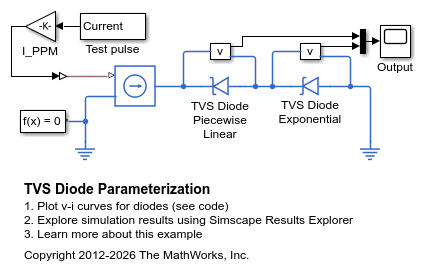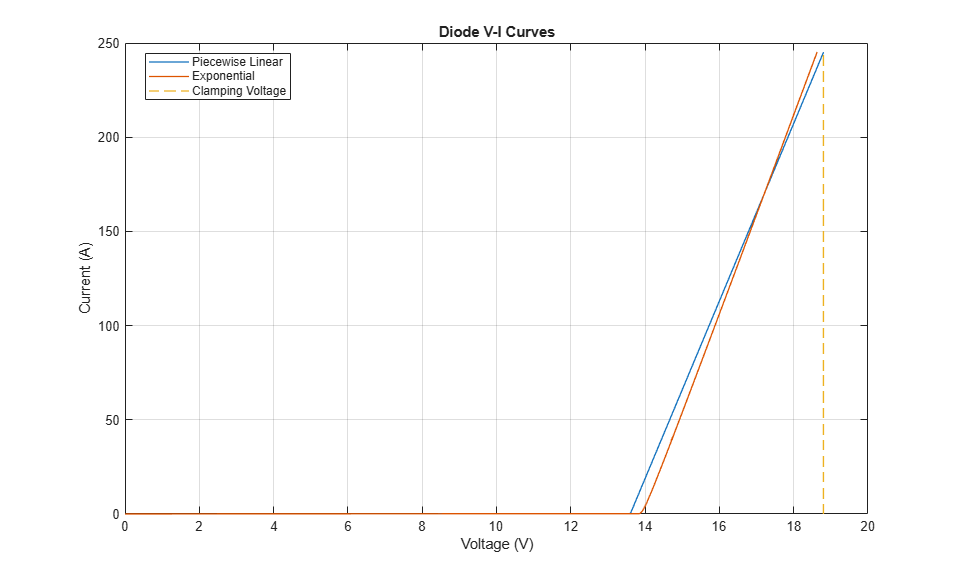# TVS Diode Parameterization

This model shows how to parameterize the Simscape™ Electrical™ diode to represent a Transient Voltage Suppression (TVS) diode. This example is for a TVS diode suited to protecting automotive electronics from voltage transients associated with turning off inductive loads. To view the data extracted from the datasheet, on the Modeling tab, in the Setup section, click Model Settings > Model Properties. On the Callbacks tab, click PreLoadFcn.

Two model options are shown here, the first using the Piecewise Linear Zener diode option, and the second using the Exponential diode option. The two diode blocks are parameterized directly in terms of the workspace datasheet values. Both models are tested here using a 10/1000us test pulse which raises the current to the peak pulse current I_PPM in 10us, and then lets it decay to I_PPM/2 in 1000us. The two scopes show that the max clamping voltage V_C of 18.8V is achieved to an acceptable tolerance.

The piecewise linear diode is the simplest to parameterize. The forward bias characteristics do not need to be accurate as a TVS diode is not operated in this region. A typical forward voltage drop of 0.6V is assumed. Given the [V_F I_F] forward bias data point, the on-resistance is given by (V_F-0.6)/I_F. The reverse bias data for the breakdown voltage V_BR and corresponding current I_T can then be used to determine the off conductance which is equal to I_T/V_BR. The diode reverse breakdown voltage, Vz, is then set equal to V_BR. Finally, zener resistance, Rz, is set so that when the current is I_PPM, the voltage is equal to the specified maximum clamping voltage V_C. A value of (V_C-V_BR)/I_PPM achieves this.

The exponential diode option models the reverse leakage current more accurately as the dependence on voltage is not assumed linear. By choosing to parameterize the exponential diode in terms of IS and N, the value of IS can be set equal to the reverse leakage current just before breakdown. The emission coefficient N is left as the default typical value of 1. Reverse breakdown voltage, BV, is set equal to V_BR. Finally, to ensure that the correct maximum clamping voltage V_C is achieved, the ohmic resistance, RS, must be appropriately set. This can either be by trial and error, or by approximate value of (V_C-V_BR-0.6)/I_PPM.

### Model### Simulation Results from Simscape Logging

The plot below shows the voltage-current curves for two different diode models. The piecewise linear model and exponential model can provide similar behavior depending upon how they are parameterized.